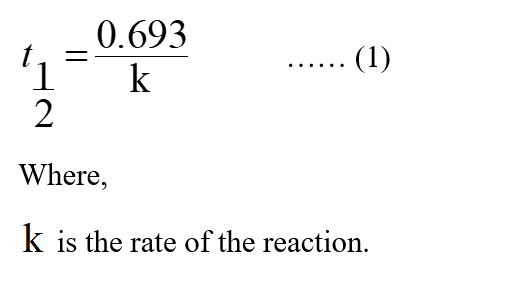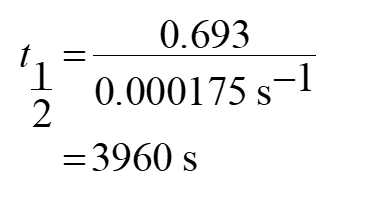# What is the half life (t1/2), in units of seconds,  for a first order reaction that has a k value of 0.000175s-1 ?

Question
9 views

What is the half life (t1/2), in units of seconds,  for a first order reaction that has a k value of 0.000175s-1 ?

check_circle

Step 1

In the first-order reaction, the half-life will not be influenced by the concentration of the substances.

Step 2

The expression to calculate the half-life for the first-order reaction is shown below:Step 3

The value of the rate of the reaction is 0.000175 s-1.

Substitute the value in equation (1).Therefore, the value of the half-life for the first-order reaction is 3960 s.

...

### Want to see the full answer?

See Solution

#### Want to see this answer and more?

Solutions are written by subject experts who are available 24/7. Questions are typically answered within 1 hour.*

See Solution
*Response times may vary by subject and question.
Tagged in